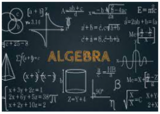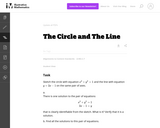Updating search results...

# 3 Results

View
Selected filters:
• UT.MATH.II.SII.MP.1Conditional Remix & Share Permitted
CC BY-NC
Rating
0.0 stars

This unit was created as an introductory/review unit for Algebra II to allow for a review of main concepts needed to progress in Algebra II. However, it could also be used in Algebra I to introduce for the first time real numbers;&nbsp;number operations;&nbsp;and equations, expressions, and inequalities.

Subject:
Secondary Mathematics
Material Type:
Homework/Assignment
Lesson
Unit of Study
Author:
Emily
02/22/2022Unrestricted Use
CC BY
Rating
0.0 stars

Although this task is fairly straightforward, it is worth noticing that it does not explicitly tell students to look for intersection points when they graph the circle and the line. Thus, in addition to assessing whether they can solve the system of equations, it is assessing a simple but important piece of conceptual understanding, namely the correspondence between intersection points of the two graphs and solutions of the system.

Subject:
Mathematics
Secondary Mathematics
Material Type:
Activity/Lab
Provider:
Illustrative Mathematics
Provider Set:
Illustrative Mathematics
Author:
Illustrative Mathematics